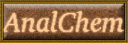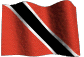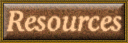### C30F Analytical chemistry labscript

A labguide has been produced for each experiment. It gives info on preparations, chemicals, apparatus and sample requirements. It also includes a helpful analytical notes feature. To get the labguide for this experiment click here Enjoy!

Back to List of Experiments

### Determination of Zn by EDTA titration and atomic absorption spectrometry

1. Introduction:

Zinc in solution may be determined by several means, including titrimetry, polarography, colorimetry and atomic absorption spectroscopy. In this experiment, zinc will be determined by two methods, namely:

(1) Titration with EDTA in alkaline solution (Vogel 1961) and (2) Flame atomic absorption spectrophotometry (FAAS).

2. Experimental Procedure:

Three (3) samples of acidified zinc solutions will be provided for analysis, labelled A, B and C. The concentrations of each solution will be provided, to allow the accuracy of the two methods to be evaluated, using statistical analyses of results generated.

(A) Titrimetry:

A standardized EDTA solution will be provided. Dilute as recommended (see Demonstrator) and use as the titrant (250mL will suffice for all titrations).

(a) Pipette three 25mL aliquots of each sample into 250mL Erlenmeyer flasks and add 5 drops of xylenol orange indicator. Add powdered hexamine, with agitation, to each yellow solution, until it changes to an intense red color.

(b) Titrate with EDTA until the color changes from red to yellow. Carry out a blank titration to determine the indicator blank, for possible correction of your sample titre values.

(c) Calculate the mean zinc concentration + std. deviation of each sample in µg/mL units.

(B) Flame atomic absorption spectrometry:

(a) Dilute each sample in triplicate with distilled water in 25mL volumetric flasks, using the dilution factors recommended (see Demonstrator).

(b) Also prepare 25mL each of a range of zinc standards (0.1, 0.5, 1.0, 1.5, and 2.0ug/mL), by appropriate dilution of the strongly acidified zinc standard provided.

(c) The atomic absorption spectrometer will be optimised in your presence. Note all the following relevant instrument operational parameters: air/acetylene flow rates, lamp current, wavelength.

(d) Note the procedures followed in the calibration of the instrument.

(e) Aspirate the Zn standards in order of increasing concentration, followed by your sample solutions, and note the respective absorbances obtained.

3. Exercises:

(A) Plot a calibration curve of absorbance vs Zn concentration. Also determine an equation for the curve using linear regression analysis. Quote the correlation coefficient.

(B) Use both of these techniques (graphical and mathematical), to determine the sample solution concentrations.

(C) Determine whether results of analyses by titrimetry and atomic absorption spectrometry (graphical and mathematical) are accurate, by statistical comparison with the reference Zn values of each sample, at the 95% confidence level (p<.05).

NB: There is no need to compare the mean values for each sample with each other in this expt.

(a) Do the results generated by the two calculation techniques used in FAAS differ significantly (p<.05) in precision with each other, and with those by titrimetry for each of the 3 samples?

(b) List 3 possible sources of error in this experiment and suggest ways of minimizing such errors.

4. Reference:

1. Vogel's Textbook of Qualitative Inorganic Analysis by Vogel, A.I., 3rd, Ed., Longman (1961) 444-445.

Anal-Chem Resources
delloyd.50megs.com
Chem. Dept. UWI. St. Augustine Campus